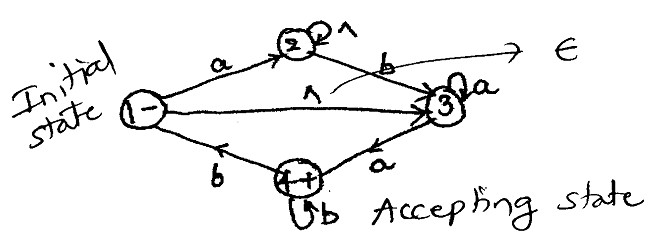MORE IN Theoretical Computer Science
MU Computer Engineering (Semester 4)
Theoretical Computer Science
December 2013
Total marks: --
Total time: --
INSTRUCTIONS
(1) Assume appropriate data and state your reasons
(2) Marks are given to the right of every question
(3) Draw neat diagrams wherever necessary

1(a) Define with example Moore and Mealy machine.
5 M
1(b) Find the equivalent DFA accepting the regular language defined by right linear grammar given as:
S$$\rightarrow$$aA|bB
A$$\rightarrow$$aA|bC|a
B$$\rightarrow$$aB|b
C$$\rightarrow$$bB
5 M
1(c) State and prove pumping lemma theorem for regular languages.
5 M
1(d) Differentiate between Deterministic PDA and Non-deterministic PDA.
5 M

2(a) Design a finite state machine to determine whether a ternary number base 3 is divisible by 5.[Hint:$$\sum$$={0,1,2}]
10 M
2(b) Design a Mealy machine for the languages (0+1)* (00+11)and convert it to a Moore machine.
10 M

3(a) Convert the following NFA with ε moves to DFA:10 M
3(b)

Let G be the grammar G={(S,X),{a,b},P,S}where production are
S$$\rightarrow$$aSX|b
X$$\rightarrow$$Xb|a
Find:
(i)Leftmost derivation (ii) Rightmost derivation and
Parse tree for the string "aababa".

10 M

4(a) Design Turing machine for the language L={anbn|n>=1}
10 M
4(b) Design a Turing machine to compare the binary numbers m and n such that if (m>n) output is G (m=n) output is E.
10 M

5(a) List and explain decision properties of regular language.
Explain the test for checking emptiness of a regular languages.
10 M
5(b) Construct left linear and right linear grammar for the regular expression:
(((01+10)*11)00)*
10 M

6(a) Construct a PDA equivalent to following grammar:
S$$\rightarrow$$OBB
B$$\rightarrow$$OS|1S|O
and show acceptance of 0104
by the PDA.
10 M
6(b) Reduce the following grammar to Greibach Normal form.
(i)S$$\rightarrow$$AB
A$$\rightarrow$$BSB|BB|b
B$$\rightarrow$$a
(ii)S$$\rightarrow$$01S|01
S$$\rightarrow$$10S|10
S$$\rightarrow$$00|^ (^ means Null (E))
10 M

Any Four
7(a) Write short notes on(any four)
Post Correspondence problem
5 M
7(b) Chomsky Hierachy
5 M
7(c) Universal turing machine
5 M
7(d) Recursive and Recursively enumerable languages
5 M
7(e) Classes of complexity.
5 M

More question papers from Theoretical Computer Science